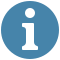## Depth first search visualization

Speed

Obstacles#### Controls

Speed - increase or decrease the speed of the visualization

Obstacles- - create walls/obstacles, on a map these can be buildings or structures which hinder a path. You can adjust the number of obstacles generated per click.
- You can also click on the grid cells to create obstacles.

Start - After adjusting the speed and creating obstacles, you can now start the visualization to see the workings of the algorithm.

Clear Path - After visualizing, you may opt to clear the path and add more obstacles.

Speed - If the grid becomes to cluttered you can reload it and repeat the above steps.

This is an empty path without any walls, you can click is to add obstacles/walls.
A

Start Node -> this is the starting point(source), search for a path starts here.
B

End Node -> where the search stops when a path to destination has been found

These act as obstacles, walls. On a real map these may be buildings or structures that may block a shorter path to a destination.
7

After the algorithm is complete, we have arrived at the destination, now the shortest path is highlighted in green with the cost of path written written in black inside the path.

### Depth first traversal.

This algorithm starts its search at the root and explores one of its children's subtree and then moves on to the next child's subtree and etcetera
The idea used is to go as deep into the graph as possible and backtrack once we reach a vertex with unvisited neighbors.
That is, start search at one vertex, after visiting the vertex, perform dfs for each unvisited adjacent vertex. In this way we visit all vertices reachable from the starting vertex.
We use a stack data structure.

Algorithm

1. Mark the current node as visited(initially current node is the root node).

2. Check if current node is the goal, If so, then return it.

1. Iterate over children nodes of current node, and do the following:

2. Check if a child node is not visited.

1.If so, then, mark it as visited.

3. Go to it's sub tree recursively until you find the goal node(In other words, do the same steps here passing the child node as the current node in the next recursive call).

4. If the child node has the goal node in this sub tree, then, return it.

3. If goal node is not found, then goal node is not in the tree!

Computational complexity

This algorithm takes O(V + E) where v is the number of vertices and e is the number of edges.

Applications

• topological sorting.
• bipartite graph testing.
• finding strongly connected components.
• solving puzzles that can only have one solution.
• detecting cycles in a graph.

References

dfs